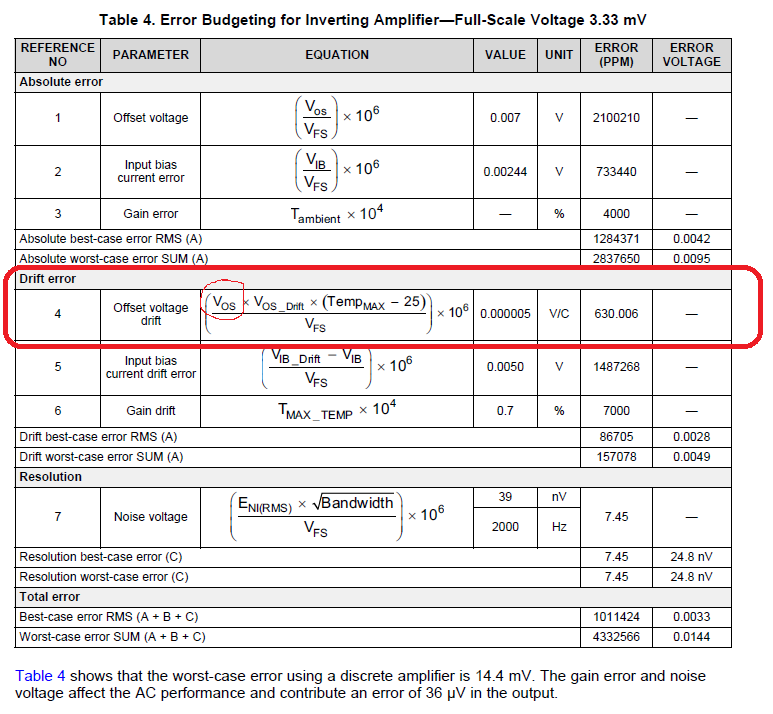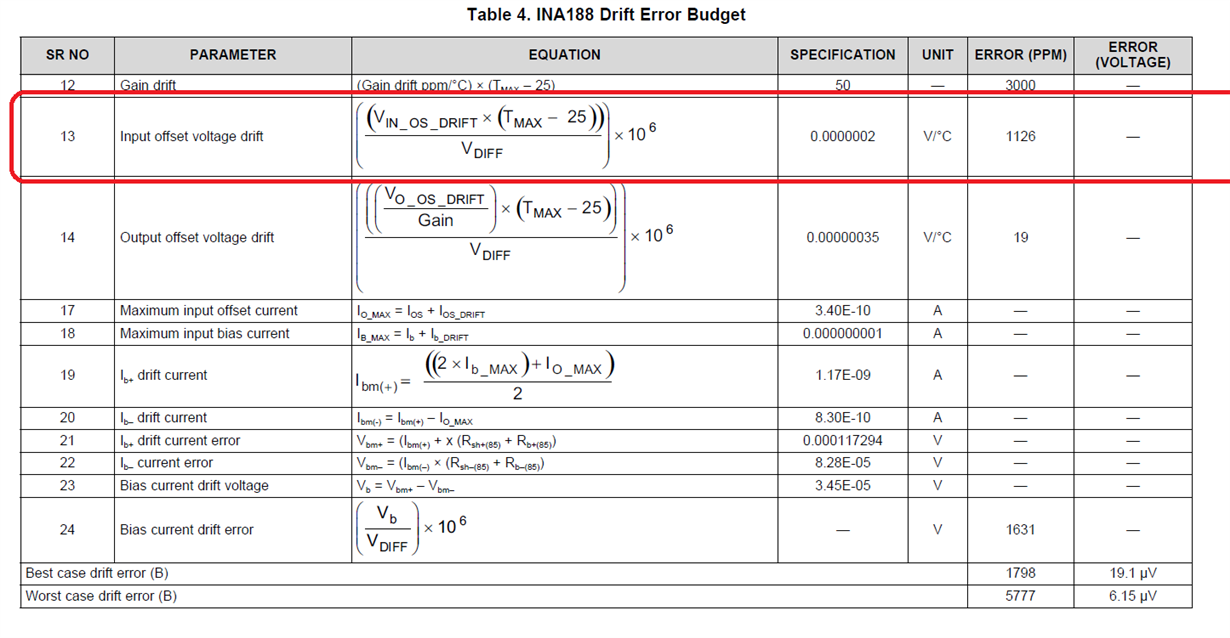If you have a related question, please click the "Ask a related question" button in the top right corner. The newly created question will be automatically linked to this question.

# TIDA-00753: Error budgeting for Inverting Amplifier - Mistake?

Part Number: TIDA-00753
Other Parts Discussed in Thread: TIDA-01063, REF3212, REF3220

Hi,

is this a mistake regarding the Voffset drift calculation in Table 4 of TIDA-00753? It shows that Voffset due to drift at Tmax should be multiplied with Vos at 25°C. Correct me if I am wrong,  but it doesn't make sense...On the other hand the calculation in TIDUBV4A has more sense...Authors for either, TIDUBV4A and TIDA-00753 are same.

Br,

Josko

• Hi,

there are some additional issue for Noise/Resolution calculation as well.

A. Although the same bandwidth is used (2000Hz), in TIDUBK3A the resolution error in Table 5 is much higher comparing the result in Table 4 for inverting amplifier in TIDA-00753. Could you check the result value in Table 4 for inverting amplifier (TIDA-00753)?

Another questions are:
1. Why the 1st order filter shaping factor 1,57 is not used in calculation for bandwidth?
2. Why are you using RMS value for noise and Vfs (full scale range) for the Resolution error calculation?
If the RMS vale is used, than the Full scale should be divided with 2*srt(2), otherwise is the Vfs actually Vpp, right?

Br,
Josko
• Thanks for sharing your queries.

I figured that a typo error resulted into incorrect equation in Table 4 of TIDA-00753.

Vos need not be multiplied,Table 4 in TIDA-01063 shows the correct equation. Thanks for highlighting i will make sure the TIDA-00753 design guide is updated with correct equation.

1) Why the 1st order filter shaping factor 1.57 is not used in calculation for bandwidth

Regarding Noise bandwidth, it depends were are we observing the noise parameter (Input of Opamp or Output of Opamp). In our calculations we are verifying effective noise within measurement bandwidth at input side of opamp to get Signal to noise ratio w.r.t CT output so we haven't multiplied gain and filter factor.

Anyway i will cross verify noise voltage value shown in Table 4 of TIDA-00753 and update the guide ASAP.

A more correct equation can be used to compute effective input noise over measurement bandwidth as shown below were eni is white noise voltage, fce is intercept frequency of 1/f and white noise curve, fh and fl are upper and lower cut off of frequency of measurement bandwidth .

Eni=eni* square root( ln(fh/fl) + (fh-fl) )

Output noise of opamp is function of opamp AC transfer function and input integrated noise Eni over measurement bandwidth.

I assume the value of 1.57 mentioned in your query corresponds to derivation of noise at opamp output stage with first order RC filter for ENB (Equivalent noise bandwidth). In case if measurement bandwidth (sampling bandwidth) is less than 3db cutoff of gain bandwidth frequency of opamp than correction needed not be considered as only fractional of aliased component of integrated noise will be present.

If SNR is computed at ADC input than output integrated noise of opamp needs to considered till sampling bandwidth.

FYI:- In TIDA-01063 we have multiplied by noise voltage factor 6 assuming 3 sigma deviation since rogowski sensor output is di/dt, so for square pulse current input we see small bipolar spikes only. Considering worst case scenario we have taken 3 sigma effect also.

2) Why are you using RMS value for noise and Vfs (full scale range) for the Resolution error calculation?

Vfs full scale voltage is RMS voltage as 100 A primary current is RMS current and with 0.1 ohm burden, 3000 turns rattio we get 3.33mV rms voltage

I hope above explanation resolves your query, in case of any more queries and doubts please don't hesitate to reach out to us.

Regards,

Srinivasan

• Hi,

Another question is related to TIDA-00753, Table 6. REF3212 Error Budgeting.
The Noise voltage for bandwidth of 5 kHz.

Could you explain, how did you get 0.000039 Value and 2206.173157 PPM error for this item related to REF3212?

The value of 0.000039 looks like 39uVrms noise from 10Hz to 10 kHz of REF3220 reference instead of 24uVrms of REF3212.

I am doing a general analysis for voltage measurement channel on HV resistor divider and trying to find out the total MAX error for REF3212 after 1 Year, and 6 Years.

BTW. Thermal hysteresis tell us about reference drift after  an excursion from room temperature to a minimum and a maximum temperature and fi nally back to room temperature.
Is there any parameter telling us about drift after soldering?

Br,

Josko

• Hi Josko,

Thanks for reaching out to us, yes you are right it is typo error it should be 24uV. (you can also use vector sum)

Based on my understanding if soldering process is followed as per TI soldering guideline the change in characteristics of device will not change as device are tested as per standards.

Regarding computing long term error drift will be tough as device is tested for 1000h.

In case if you are looking for non calibrated guaranteed accuracy/precision specs for continuous operation than it will be tough to find for 1yr/6yr.

I would suggest to have auto calibrate in software every 1000 hrs to maintain guaranteed specs by having RTC in system and measuring voltage during no operation mode.

Regards,

Srinivasan